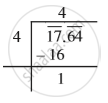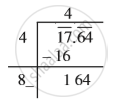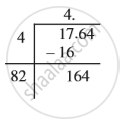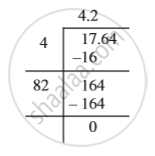Share

# Square Roots of Decimals

#### notes

Consider sqrt 17.64
Step 1:  To find the square root of a decimal number we put bars on the integral part (i.e., 17) of the number in the usual manner. And place bars on the decimal part (i.e., 64) on every pair of digits beginning with the first decimal place. Proceed as usual. We get
bar 17.bar 64.

Step 2 :
Now proceed in a similar manner. The left most bar is on 17 and 4^2 < 17 < 5^2. Take this number as the divisor and the number under the left-most bar as the dividend, i.e., 17. Divide and get the remainder.Step 3: The remainder is 1. Write the number under the next bar (i.e., 64) to the right of this remainder, to get 164.Step 4: Double the divisor and enter it with a blank on its right. Since 64 is the decimal part so put a decimal point in the quotient.Step 5: We know 82 × 2 = 164, therefore, the new digit is 2. Divide and get the remainder.Step 6: Since the remainder is 0 and no bar left, therefore sqrt 17.64  = 4.2 .

S